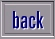Home Page Biology Chemistry Physics Science Superstars Inventions Teachers Stress Homework Help Zephyrus Training About Us Contact Us Credits Disclaimer Privacy Cookies

 Question: Please can you give me an explanation about square numbers and square roots and about cube roots. It would be helpful if you could also give a few examples. Answer: 1. Square Numbers: To find the square of a number, you just multiply it by itself, so: a. 4 squared = 4 x 4 = 16 b. 12 squared = 12 x 12 = 144 c. -7 squared = -7 x -7 = 49 (Remember the rule that a minus x a minus gives a positive number) 2. Square Roots: These are the opposite of squares, so you are looking at the two numbers that when multiplied together give you the number you start with. A number can have both positive and negative square roots. (Remember the rule that a minus x a minus gives a positive number) a. Square roots of 64 are +8 and -8. (8 x 8 = 64 and -8 x -8 = 64) b. Square roots of 121 are +11 and -11 c. Square roots of 0.25 are +0.5 and -0.5 d. Square roots of 100 are +10 and -10 3. Cube Numbers: To find the cube of a number, multiply the number by itself and then by itself again, so: a. 3 cubed = 3 x 3 x 3 = 27 b. 5 cubed = 5 x 5 x 5 = 125 c. -7 cubed = -7 x -7 x -7 = -343 (Watch these - when you square two negative numbers it becomes positive, but when you multiply by another negative, it becomes negative again.)to homework page